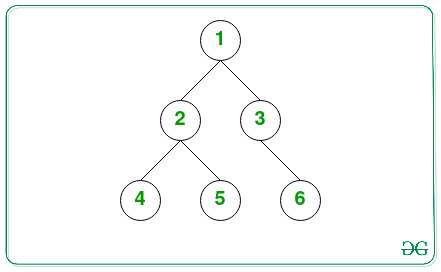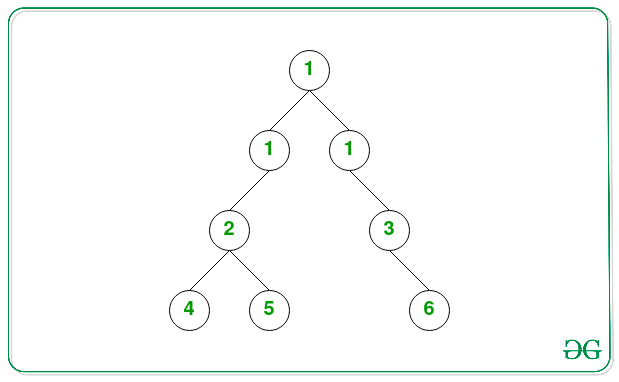Skip to content
Related Articles
Modify a Binary Tree by adding a level of nodes with given value at a specified level
• Difficulty Level : Hard
• Last Updated : 28 Apr, 2021

Given a Binary Tree consisting of N nodes and two integers K and L, the task is to add one row of nodes of value K at the Lth level, such that the orientation of the original tree remains unchanged.

Examples:

Input: K = 1, L = 2Output:
1
1 1
2 3
4 5 6
Explanation:
Below is the tree after inserting node with value 1 in the K(= 2) th level.Input: K = 1, L = 1Output:
1
1
2 3
4 5 6

Approach: The given problem can be solved by using Breadth First search for traversal of the tree and adding nodes with a given value between a node at level (L – 1) and roots of its left and right subtree. Follow the steps below to solve the problem:

• If L is 1 then make the new node with value K then join the current root to the left of the new node making the new node the root node.
• Initialize a Queue, say Q which is used to traverse the tree using BFS.
• Initialize a variable, say CurrLevel that stores the current level of a node.
• Iterate while Q is not empty() and CurrLevel is less than (L – 1) and perform the following steps:
• Store the size of queue Q in a variable say len.
• Iterate while len is greater than 0 and then pop the front element of the queue and push the left and the right subtree in Q.
• Increment the value of CurrLevel by 1.
• Now again iterate while Q is not empty() and perform the following steps:
• Store the front node of Q in a variable say temp and pop the front element.
• Store the left and the right subtree of temp node in variables, say temp1 and temp2 respectively.
• Create a new node with value K and then join the current node to the left of node temp by assigning the node value to temp.left.
• Again create a new node with value K and then join the current node to the right of node temp by assigning the node value to temp.right.
• Then join the temp1 to the left of the new node i.e., temp.left.left and temp2 to the right of the new node i.e., temp.right.right.
• After completing the above steps, print the tree in level order traversal.

Below is the implementation of the above approach:

## C++

 `// C++ program for the above approach``#include ` `using` `namespace` `std;` `// Class of TreeNode``struct` `TreeNode {``    ``int` `val;``    ``TreeNode *left;``    ``TreeNode *right;``   ` `  ``// Constructor``    ``TreeNode(``int` `v)``    ``{``        ``val = v;``        ``left = right = NULL;``    ``}``};` `// Function to add one row to a``// binary tree``TreeNode *addOneRow(TreeNode *root, ``int` `K, ``int` `L)``{``    ``// If L is 1``    ``if` `(L == 1) {` `        ``// Store the node having``        ``// the value K``        ``TreeNode *t = ``new` `TreeNode(K);` `        ``// Join node t with the``        ``// root node``        ``t->left = root;``        ``return` `t;``    ``}` `    ``// Stores the current Level``    ``int` `currLevel = 1;` `    ``// For performing BFS traversal``    ``queue Q;` `    ``// Add root node to Queue Q``    ``Q.push(root);` `    ``// Traversal while currLevel``    ``// is less than L - 1``    ``while` `(Q.size() > 0 && currLevel < L - 1)``    ``{` `        ``// Stores the count of the``        ``// total nodes at the``        ``// currLevel``        ``int` `len = Q.size();` `        ``// Iterate while len``        ``// is greater than 0``        ``while` `(len > 0)``        ``{` `            ``// Pop the front``            ``// element of Q``            ``TreeNode *node = Q.front();``            ``Q.pop();` `            ``// If node.left is``            ``// not NULL``            ``if` `(node->left != NULL)``                ``Q.push(node->left);` `            ``// If node.right is``            ``// not NULL``            ``if` `(node->right != NULL)``                ``Q.push(node->right);` `            ``// Decrement len by 1``            ``len--;``        ``}` `        ``// Increment currLevel by 1``        ``currLevel++;``    ``}` `    ``// Iterate while Q is``    ``// non empty()``    ``while` `(Q.size() > 0)``    ``{` `        ``// Stores the front node``        ``// of the Q queue``        ``TreeNode *temp = Q.front();``        ``Q.pop();` `        ``// Stores its left sub-tree``        ``TreeNode *temp1 = temp->left;` `        ``// Create a new Node with``        ``// value K and assign to``        ``// temp.left``        ``temp->left = ``new` `TreeNode(K);` `        ``// Assign temp1 to the``        ``// temp.left.left``        ``temp->left->left = temp1;` `        ``// Store its right subtree``        ``TreeNode *temp2 = temp->right;` `        ``// Create a new Node with``        ``// value K and assign to``        ``// temp.right``        ``temp->right = ``new` `TreeNode(K);` `        ``// Assign temp2 to the``        ``// temp.right.right``        ``temp->right->right = temp2;``    ``}` `    ``// Return the updated root``    ``return` `root;``}` `// Function to print the tree in``// the level order traversal``void` `levelOrder(TreeNode *root)``{``    ``queue Q;` `    ``if` `(root == NULL) {``        ``cout<<(``"Null"``)< 0) {` `        ``// Stores the total nodes``        ``// at current level``        ``int` `len = Q.size();` `        ``// Iterate while len``        ``// is greater than 0``        ``while` `(len > 0) {` `            ``// Stores the front Node``            ``TreeNode *temp = Q.front();``            ``Q.pop();` `            ``// Print the value of``            ``// the current node``            ``cout << temp->val << ``" "``;` `            ``// If reference to left``            ``// subtree is not NULL``            ``if` `(temp->left != NULL)` `                ``// Add root of left``                ``// subtree to Q``                ``Q.push(temp->left);` `            ``// If reference to right``            ``// subtree is not NULL``            ``if` `(temp->right != NULL)` `                ``// Add root of right``                ``// subtree to Q``                ``Q.push(temp->right);` `            ``// Decrement len by 1``            ``len--;``        ``}` `        ``cout << endl;``    ``}``}` `// Driver Code``int` `main()``{``  ` `    ``// Given Tree``    ``TreeNode *root = ``new` `TreeNode(1);``    ``root->left = ``new` `TreeNode(2);``    ``root->left->left = ``new` `TreeNode(4);``    ``root->left->right = ``new` `TreeNode(5);``    ``root->right = ``new` `TreeNode(3);``    ``root->right->right = ``new` `TreeNode(6);` `    ``int` `L = 2;``    ``int` `K = 1;` `    ``levelOrder(addOneRow(root, K, L));``}` `// This code is contributed by mohit kumar 29.`

## Java

 `// Java program for the above approach` `import` `java.io.*;``import` `java.util.*;` `class` `GFG {` `    ``// Class of TreeNode``    ``public` `static` `class` `TreeNode {``        ``int` `val;``        ``TreeNode left;``        ``TreeNode right;``        ``TreeNode() {}` `        ``// Constructor``        ``TreeNode(``int` `val)``        ``{``            ``this``.val = val;``        ``}``    ``}` `    ``// Function to add one row to a``    ``// binary tree``    ``public` `static` `TreeNode addOneRow(``        ``TreeNode root, ``int` `K, ``int` `L)``    ``{``        ``// If L is 1``        ``if` `(L == ``1``) {` `            ``// Store the node having``            ``// the value K``            ``TreeNode t = ``new` `TreeNode(K);` `            ``// Join node t with the``            ``// root node``            ``t.left = root;``            ``return` `t;``        ``}` `        ``// Stores the current Level``        ``int` `currLevel = ``1``;` `        ``// For performing BFS traversal``        ``Queue Q``            ``= ``new` `LinkedList();` `        ``// Add root node to Queue Q``        ``Q.add(root);` `        ``// Traversal while currLevel``        ``// is less than L - 1``        ``while` `(!Q.isEmpty()``               ``&& currLevel < L - ``1``) {` `            ``// Stores the count of the``            ``// total nodes at the``            ``// currLevel``            ``int` `len = Q.size();` `            ``// Iterate while len``            ``// is greater than 0``            ``while` `(len > ``0``) {` `                ``// Pop the front``                ``// element of Q``                ``TreeNode node = Q.poll();` `                ``// If node.left is``                ``// not null``                ``if` `(node.left != ``null``)``                    ``Q.add(node.left);` `                ``// If node.right is``                ``// not null``                ``if` `(node.right != ``null``)``                    ``Q.add(node.right);` `                ``// Decrement len by 1``                ``len--;``            ``}` `            ``// Increment currLevel by 1``            ``currLevel++;``        ``}` `        ``// Iterate while Q is``        ``// non empty()``        ``while` `(!Q.isEmpty()) {` `            ``// Stores the front node``            ``// of the Q queue``            ``TreeNode temp = Q.poll();` `            ``// Stores its left sub-tree``            ``TreeNode temp1 = temp.left;` `            ``// Create a new Node with``            ``// value K and assign to``            ``// temp.left``            ``temp.left = ``new` `TreeNode(K);` `            ``// Assign temp1 to the``            ``// temp.left.left``            ``temp.left.left = temp1;` `            ``// Store its right subtree``            ``TreeNode temp2 = temp.right;` `            ``// Create a new Node with``            ``// value K and assign to``            ``// temp.right``            ``temp.right = ``new` `TreeNode(K);` `            ``// Assign temp2 to the``            ``// temp.right.right``            ``temp.right.right = temp2;``        ``}` `        ``// Return the updated root``        ``return` `root;``    ``}` `    ``// Function to print the tree in``    ``// the level order traversal``    ``public` `static` `void` `levelOrder(``        ``TreeNode root)``    ``{``        ``Queue Q``            ``= ``new` `LinkedList<>();` `        ``if` `(root == ``null``) {``            ``System.out.println(``"Null"``);``            ``return``;``        ``}` `        ``// Add root node to Q``        ``Q.add(root);` `        ``while` `(!Q.isEmpty()) {` `            ``// Stores the total nodes``            ``// at current level``            ``int` `len = Q.size();` `            ``// Iterate while len``            ``// is greater than 0``            ``while` `(len > ``0``) {` `                ``// Stores the front Node``                ``TreeNode temp = Q.poll();` `                ``// Print the value of``                ``// the current node``                ``System.out.print(``                    ``temp.val + ``" "``);` `                ``// If reference to left``                ``// subtree is not null``                ``if` `(temp.left != ``null``)` `                    ``// Add root of left``                    ``// subtree to Q``                    ``Q.add(temp.left);` `                ``// If reference to right``                ``// subtree is not null``                ``if` `(temp.right != ``null``)` `                    ``// Add root of right``                    ``// subtree to Q``                    ``Q.add(temp.right);` `                ``// Decrement len by 1``                ``len--;``            ``}` `            ``System.out.println();``        ``}``    ``}` `    ``// Driver Code``    ``public` `static` `void` `main(String[] args)``    ``{``        ``// Given Tree``        ``TreeNode root = ``new` `TreeNode(``1``);``        ``root.left = ``new` `TreeNode(``2``);``        ``root.left.left = ``new` `TreeNode(``4``);``        ``root.left.right = ``new` `TreeNode(``5``);``        ``root.right = ``new` `TreeNode(``3``);``        ``root.right.right = ``new` `TreeNode(``6``);` `        ``int` `L = ``2``;``        ``int` `K = ``1``;` `        ``levelOrder(addOneRow(root, K, L));``    ``}``}`
Output:
```1
1 1
2 3
4 5 6```

Time Complexity: O(N)
Auxiliary Space: O(N)

Attention reader! Don’t stop learning now. Get hold of all the important DSA concepts with the DSA Self Paced Course at a student-friendly price and become industry ready.  To complete your preparation from learning a language to DS Algo and many more,  please refer Complete Interview Preparation Course.

In case you wish to attend live classes with industry experts, please refer DSA Live Classes

My Personal Notes arrow_drop_up